# Worked example of Kuzmin-Turek stabilization

This page is part of a set of pages devoted to discussions of numerical stabilization in PorousFlow. See:

Kuzmin and Turek (Kuzmin and Turek, 2004) describe a method of stabilising advection while minimising artificial numerical diffusion. In this page "Kuzmin and Turek" is abbreviatved to "KT". This page will make much more sense if you read it in tandem with the KT paper! KT consider a single scalar quantity that is being advected. For sake of argument, in this page we think of as "heat".

In this page, the 1D example studied in the numerical diffusion page is used to explicitly illustrate how their approach works. The input file is

# Using Flux-Limited TVD Advection ala Kuzmin and Turek, with antidiffusion from superbee flux limiting
[Mesh]
type = GeneratedMesh
dim = 1
nx = 100
xmin = 0
xmax = 1
[]

[Variables]
[./tracer]
[../]
[]

[ICs]
[./tracer]
type = FunctionIC
variable = tracer
function = 'if(x<0.1,0,if(x>0.3,0,1))'
[../]
[]

[Kernels]
[./mass_dot]
type = MassLumpedTimeDerivative
variable = tracer
[../]
[./flux]
variable = tracer
[../]
[]

[UserObjects]
[./fluo]
flux_limiter_type = superbee
u = tracer
velocity = '0.1 0 0'
[../]
[]

[BCs]
[./no_tracer_on_left]
type = PresetBC
variable = tracer
value = 0
boundary = left
[../]
[./remove_tracer]
# Ideally, an OutflowBC would be used, but that does not exist in the framework
# In 1D VacuumBC is the same as OutflowBC, with the alpha parameter being twice the velocity
type = VacuumBC
boundary = right
alpha = 0.2 # 2 * velocity
variable = tracer
[../]
[]

[Preconditioning]
active = basic
[./basic]
type = SMP
full = true
petsc_options = '-ksp_diagonal_scale -ksp_diagonal_scale_fix'
petsc_options_iname = '-pc_type -sub_pc_type -sub_pc_factor_shift_type -pc_asm_overlap'
petsc_options_value = ' asm      lu           NONZERO                   2'
[../]
[./preferred_but_might_not_be_installed]
type = SMP
full = true
petsc_options_iname = '-pc_type -pc_factor_mat_solver_package'
petsc_options_value = ' lu       mumps'
[../]
[]

[VectorPostprocessors]
[./tracer]
type = LineValueSampler
start_point = '0 0 0'
end_point = '1 0 0'
num_points = 101
sort_by = x
variable = tracer
[../]
[]

[Executioner]
type = Transient
solve_type = Newton
end_time = 6
dt = 6E-2
nl_abs_tol = 1E-8
nl_max_its = 500
timestep_tolerance = 1E-3
[]

[Outputs]
[./out]
type = CSV
execute_on = final
[../]
[]

(modules/porous_flow/test/tests/numerical_diffusion/fltvd.i)

The mesh sits in the domain and is meshed with 100 elements. The initial condition is if , and otherwise. The velocity is uniform to the right: .

The key differences between this input file and any other that simulated advection is the use of the FluxLimitedTVDAdvection Kernel and the AdvectiveFluxCalculatorConstantVelocity UserObject. The latter computes , and that are used by the Kernel as described in detail in this page.

The above input file sets the flux_limiter_type = superbee, but different types, such as none, vanleer, minmod or mc may be chosen. As explained in detail below, these add antidiffusion to counteract the artificial numerical diffusion added to stabilize the problem (except for the none choice that adds no antidiffusion).

KT work with a lumped mass matrix (see discussion at the start of KT Section 5, Eqn (25)), so the input file uses the MassLumpedTimeDerivative Kernel.

## No stabilization

Without any stabilization, the KT method is just the usual Galerkin finite-element method used by default in MOOSE. The advection equation (1) is discretised (employing mass-lumping) to (2) where is the lumped mass at node and is the value of at node . In this equation, KT have introduced the transport matrix, , with entries (3) Here is the test function at node and is the advective velocity at node . See KT Eqns (18), (19), (20), (25), (26) and (31). The residual of the advection term at node is (4) See KT Eqn (31).

is evaluated for all pairs of nodes that are linked by the mesh. Therefore, it may be evaluated by looping over elements, and adding contributions to the appropriate - entries. This is done by the AdvectiveFluxCalculatorConstantVelocity UserObject.

Let us evaluate for the initial configuration of our test example. Each element yields (5) In this equation, the and subscripts mean "node to the left" and "node to the right". Summing up all such contributions yields (6) The top-left entry and the bottom-right entry may be safely ignored in the subsequent presentation, as they will be influenced by any boundary conditions that are on the left and right sides, respectively.

Inserting Eq. (6) into Eq. (2) leads to the following standard central-difference representation of the advection equation: (7) (The equation will be different at the mesh boundaries, depending on the boundary conditions.)

Now consider the node at the position of the front (at ). It has , while . This means that . For implicit timestepping, the explicit solution of Eq. (7) is not immediately obvious, but nonetheless, the crucial point remains: spurious overshoots and undershoots appear in the solution, as shown pictorially in Figure 1.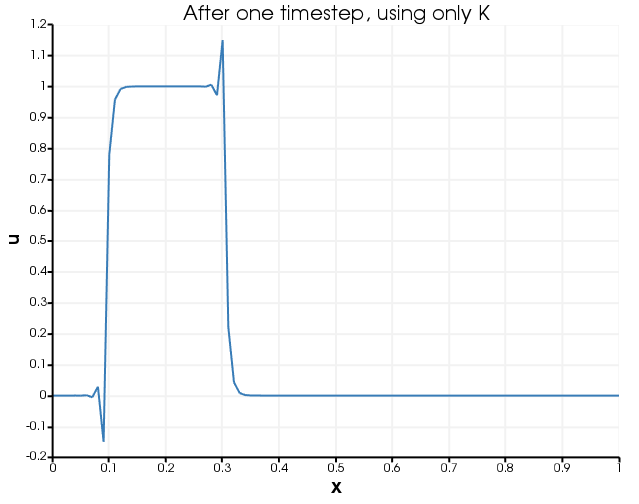Figure 1: Temperature profile after one timestep, when only is used to transport the heat. Notice the overshoots and undershoots.

So far, the presentation has invoved only the standard Galerkin finite element scheme, with no special input from KT (other than their notation and referring to their formulae). Now let us slowly introduce KT's design.

## Stabilization using upwinding

To remove the spurious oscillations that result from using to transport , KT note that it is the negative off-diagonal entries of that are problematic. In our example this is clear: we want to remove them so the discretised central-difference equation Eq. (7) becomes a full upwind equation: (8) Here and below it is assumed , otherwise the upwinding goes the opposite way, that is, from node to node .

KT eliminate these negative off-diagonal entries in the following way. Introduce the symmetric matrix , with entries (9) (KT Eqn (32)). So:

• picks out the negative off-diagonal entries of , so it contains the entries that we want to elimiate

• is diagonal and each row of sums to zero, hence is a discrete diffusion operator

In our case (10)

Then, instead of , the operator is used to transport : (11) In our case (12) (Once again, the top-left and bottom-right entries are potentially modified by boundary conditions.) The full-upwind equation, Eq. (8), is evidently obtained.

KT demonstrate that this process produces an that is Locally Extremum Diminishing, ie, that it never increases oscillations in the temperature profile. Unfortunately, the process of employing instead of has (by design) added diffusion (), and this is evident in the results, see Figure 2.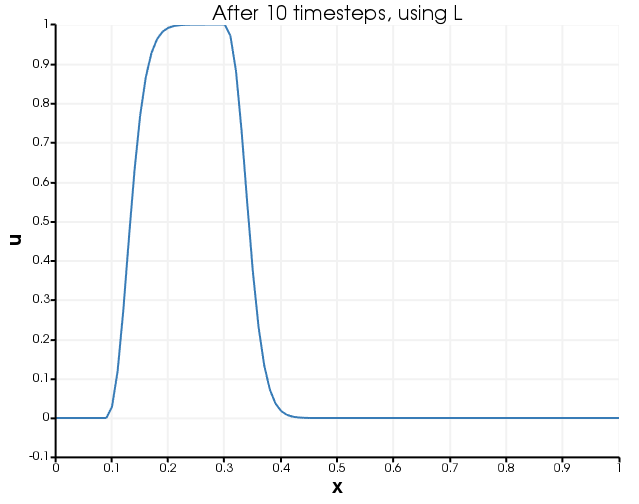Figure 2: Temperature profile after one timestep, when operator is used to transport the heat. There are no overshoots and undershoots, but the sharp profile has diffused.

## Flux-limiting and anti-diffusion

It is good to add diffusion () around the front position, because it prevents overshoots and undershoots, but elsewhere it is disasterous because it results in unphysical diffusion. Therefore, KT add some anti-diffusion in regions where is not needed, to counter the effect of . KT introduce and (Eqns (46), (47) and (48)) defined by (13) and (14) In our case, using Eq. (6), these are (15) and (16) Note that concerns the negative entries of , so these are the bits that are removed by adding diffusion . On the other hand, is harmless: these parts do not introduce any overshoots or undershoots. KT argue that looking at the ratio should enable us to determine how much of really needed to be eliminated.

To this end, KT split and into their positive and negative parts, and limit these parts separately (see Eqns (47), (48), (49) and the discussion of flux limiting on pp 134–135). In our simple situation, there are four distinct cases, that are explicitly worked out in Table 1. In working through this table, note that the end goal is (defined in KT Eqn (50)), which is the antidiffusive flux travelling from downwind node to the upwind node . Its purpose is to counter the diffusion we added in the matrix.

Table 1: Possible choices for u around node and the consequences for the antidiffusion. A "?" indicates the quantity is ill-defined due to a division , but that the quantity does not matter. In each case node is downwind of node .

and and and and
at maximum increasing downstream at minimum decreasing downstream
00
00
00
00
0??
?0?
0 (by )0 (by )

The antidiffusive flux is zero when node is at a maximum or a minimum. This is physically motivated by the "LED" criteria: we don't want local extrema to become more extreme: instead, we want to smooth them away with diffusion. However, the antidiffusive flux is nonzero when the values of around node are such that is simply increasing or decreasing. In these cases the addition of diffusion is not necessary, and the antidiffusion counters (to some extent, depending on ).

The controlling factor is the flux limiter, . Note that it is a function of the surrounding values of : (17) This is described in KT Figure 1. Note that it is exactly the flux-limiter used in RDG(P0P1) (see the RDG page), so identical results to RDG(P0P1) should be expected in simple situations.

It is possible to choose all sorts of functional forms for . KT write 4 possibilities on page 135. All these possibilities satisfy . This means that the antidiffusive flux will always be (18) With this antidiffusive flux, the evolution equation (see Step 3 of KT's Fig 2, along with Eqn(36) and the final expression in Eqn(50)) is just (19) This is the final result of KT's procedure.

When , KT's procedure yields the full upwind equation Eq. (8). When , KT's procedure yields the central difference equation Eq. (7). When , KT's procedure yields the downwind equation . In general practice, KT's procedure produces no spurious overshoots or undershoots while strongly limiting artificial numerical diffusion. The result for our test case is shown in Figure 3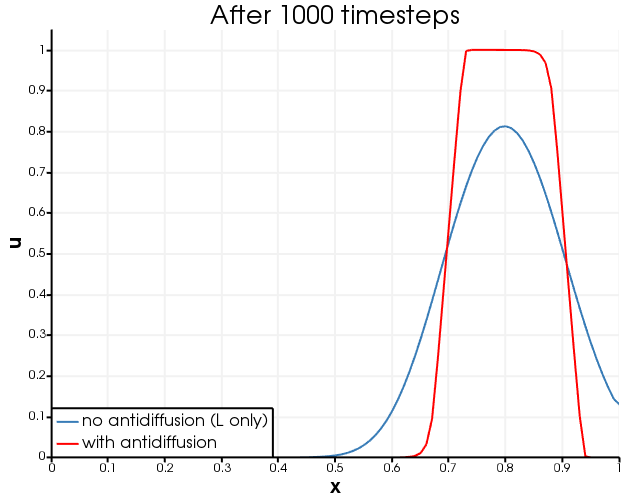Figure 3: Temperature profile after 1000 timesteps, when operator is used to transport the heat, and when plus antidiffusion is used.

## Flux limiters

KT present four different flux-limiters. These are plotted in Figure 4.

Notice that the VanLeer limiter is smoother than the others, so it is likely to provide superior nonlinear concergence, and it is chosen as the default limiter in the AdvectiveFluxCalculatorConstantVelocity.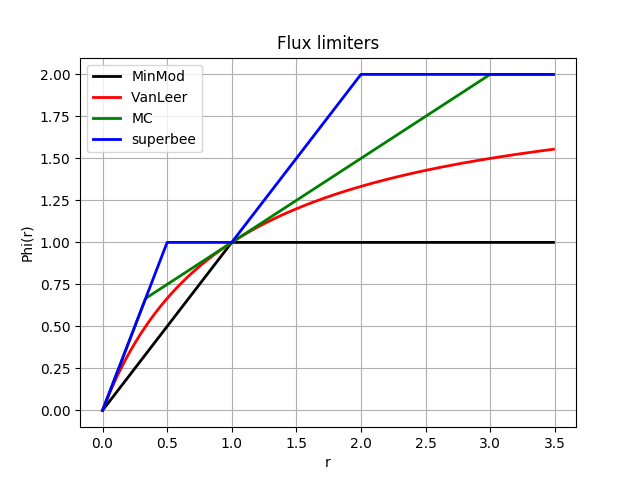Figure 4: Flux limiters, , enumerated by KT (pp 135).

## Effect of different flux limiters

The effect of choosing different flux limiters for this worked example is shown in Figure 5. In this case, the superbee flux limiter performs best. Note that the timestep is rather large in this example, to emphasize the differences between the flux limiters, however, if this were a real simulation that was trying to reduce numerical diffusion, the timestep should be chosen smaller, and then the results would look more like Figure 3.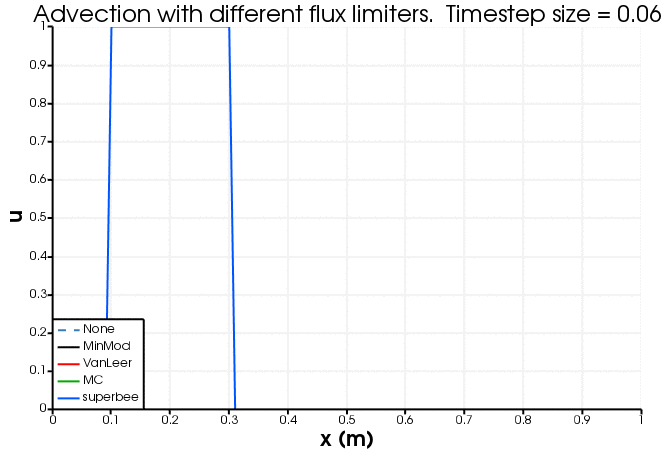Figure 5: The effect of flux limiter on the advective profile. Note that the timestep is rather large here, to emphasise the difference between the flux limiters. If a smaller timestep is chosen the results all look similar and like Figure 3.

1. D. Kuzmin and S. Turek. High-resolution FEM-TVD shcemes based on a fully multidimensional flux limiter. Journal of Computational Physics, 198:131–158, 2004.[BibTeX]